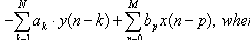## Digital system equationBack to the DSP thread. Let’s talk about the digital system equation. Every digital system can be described using this equation. The output of the digital filter generally consists of previous inputs and previous outputs. y(n-k) is considered as previous outputs; x(n-p) – previous inputs; ak and b­p – coefficients; This equation is convenient to define the discrete systems and extract various characteristics. Number N defines a discrete filter tap (Tap – A FIR “tap” is simply a coefficient/delay pair). Lets say we have digital filter made of one tap. y(n)=a·y(n-1)+b·x(n) First we calculate filter response function – h(n): Response function is calculated from system reaction to discrete impulse ´(n). So assume that x(n)= ´(n) and y(n)=h(n). Initial conditions: y(-1)=h(-1)=0. Then: h(n)=0 when n<0; h(0)=a·h(-1)+b·´(n)=b; h(1)=a·h(0)+b·´(1)=ab; ……. h(n)= ban where n>0 Lets say we have a=0.7; b=1; Then we get discrete system characteristics: This equation in example describes IIR (Infinite Impulse Response) discrete systems because there is a feedback element a·y(n-1). There is another type of discrete filter – FIR (Finite Impulse Response) Finite response filter equation is much simpler: As you may notice from the equation – the IIR filter has an infinite number of response impulses while the…